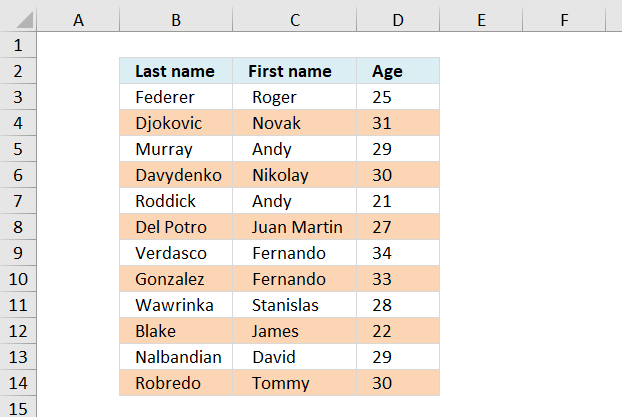Author: Oscar Cronquist Article last updated on June 03, 2022Here is how to highlight every other row using conditional formatting.

Conditional formatting formula:

=ISEVEN(ROW())*OR(\$B3:\$D3<>"")

Alternative CF formula:

=EVEN(ROW())=ROW()

This formula colors every second row if any cell in the row is populated. If you know you will add more records later to the list, select and conditional format a range larger than the list. Empty rows won´t be formatted.

How to apply the conditional formatting formula in excel 2007:

1. Select the range, example cell range A:C
2. Press with left mouse button on "Home" tab on the ribbon
3. Press with left mouse button on "Conditional formatting"
4. Press with left mouse button on "New rule..."
5. Press with left mouse button on "Use a formula to determine which cells to format"
6. Press with left mouse button on "Format values where this formula is true" window.
7. Type =ISEVEN(ROW())*OR(\$B3:\$D3<>"")
8. Press with left mouse button on Format button
9. Press with left mouse button on "Fill" tab
10. Select a color
11. Press with left mouse button on OK!
12. Press with left mouse button on OK!

### Explaining the contional formatting formula in cell A2

=ISEVEN(ROW())*OR(\$A2:\$C2<>"")

Step 1 - Check if row number is even

=ISEVEN(ROW())*OR(\$A2:\$C2<>"")

ISEVEN(ROW())

becomes

ISEVEN(2)

returns TRUE in cell A2.

Step 2 - Check if  any of the cells in row are not empty

=ISEVEN(ROW())*OR(\$A2:\$C2<>"")

OR(\$A2:\$C2<>"")

becomes

OR({"Federer", " Roger ", 25}<>"")

becomes

OR({TRUE, TRUE, TRUE})

and returns TRUE

Step 3 - Multiply functions (AND logic)

Both functions need to return TRUE in order to return TRUE

=ISEVEN(ROW())*OR(\$A2:\$C2<>"")

becomes

TRUE*TRUE

and returns TRUE. Row 2 is conditional formatted.

### Get excel sample file for this tutorial

color-every-second-row-using-dynamic-conditional-formatting.xls
(Excel 97-2003 Workbook *.xls)